# Alternating Current (AC) Introduction

Alternating current (AC) electrical systems are found on most multi-engine, high performance turbine powered aircraft and transport category aircraft. AC is the same type of electricity used in industry and to power our homes. Direct current (DC) is used on systems that must be compatible with battery power, such as on light aircraft and automobiles. There are many benefits of AC power when selected over DC power for aircraft electrical systems.

AC can be transmitted over long distances more readily and more economically than DC, since AC voltages can be increased or decreased by means of transformers. Because more and more units are being operated electrically in airplanes, the power requirements are such that a number of advantages can be realized by using AC (especially with large transport category aircraft). Space and weight can be saved since AC devices, especially motors, are smaller and simpler than DC devices. In most AC motors, no brushes are required, and they require less maintenance than DC motors. Circuit breakers operate satisfactorily under loads at high altitudes in an AC system, whereas arcing is so excessive on DC systems that circuit breakers must be replaced frequently. Finally, most airplanes using a 24-volt DC system have special equipment that requires a certain amount of 400 cycle AC current. For these aircraft, a unit called an inverter is used to change DC to AC.

AC is constantly changing in value and polarity, or as the name implies, alternating. Figure 1 shows a graphic comparison of DC and AC.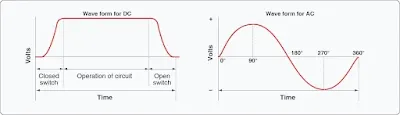Figure 1. DC and AC voltage curves

The polarity of DC never changes, and the polarity and voltage constantly change in AC. It should also be noted that the AC cycle repeats at given intervals. With AC, both voltage and current start at zero, increase, reach a peak, then decrease and reverse polarity. If one is to graph this concept, it becomes easy to see the alternating wave form. This wave form is typically referred to as a sine wave.

## Definitions

### Values of AC

There are three values of AC that apply to both voltage and current. These values help to define the sine wave and are called instantaneous, peak, and effective. It should be noted that during the discussion of these terms, the text refers to voltage. But remember, the values apply to voltage and current in all AC circuits.

### Instantaneous

An instantaneous voltage is the value at any instant in time along the AC wave. The sine wave represents a series of these values. The instantaneous value of the voltage varies from zero at 0° to maximum at 90°, back to zero at 180°, to maximum in the opposite direction at 270°, and to zero again at 360°. Any point on the sine wave is considered the instantaneous value of voltage.

### Peak

The peak value is the largest instantaneous value, often referred to as the maximum value. The largest single positive value occurs after a certain period of time when the sine wave reaches 90°, and the largest single negative value occurs when the wave reaches 270°. Although important in the understanding of the AC sine wave, peak values are seldom used by aircraft technicians.

### Effective

The effective values for voltage are always less than the peak (maximum) values of the sine wave and approximate DC voltage of the same value. For example, an AC circuit of 24 volts and 2 amps should produce the same heat through a resistor as a DC circuit of 24 volts and 2 amps. The effective value is also known as the root mean square, or RMS value, which refers to the mathematical process by which the value is derived.

Most AC meters display the effective value of the AC. In almost all cases, the voltage and current ratings of a system or component are given in effective values. In other words, the industry ratings are based on effective values. Peak and instantaneous values, used only in very limited situations, would be stated as such. In the study of AC, any values given for current or voltage are assumed to be effective values unless otherwise specified. In practice, only the effective values of voltage and current are used.

The effective value is equal to .707 times the peak (maximum) value. Conversely, the peak value is 1.41 times the effective value. Thus, the 110 volt value given for AC is only 0.707 of the peak voltage of this supply. The maximum voltage is approximately 155 volts (110 × 1.41 = 155 volts maximum).

How often the AC waveform repeats is known as the AC frequency. The frequency is typically measured in cycles per second (CPS) or hertz (Hz). One Hz equals one CPS. The time it takes for the sine wave to complete one cycle is known as period (P). Period is a value or time period and typically measured in seconds, milliseconds, or microseconds. It should be noted that the time period of a cycle can change from one system to another; it is always said that the cycle completes in 360° (related to the 360° of rotation of an AC alternator). [Figure 2]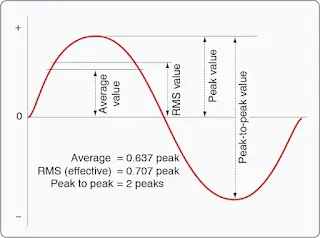Figure 2. Values of AC

### Cycle Defined

A cycle is a completion of a pattern. Whenever a voltage or current passes through a series of changes, returns to the starting point, and then repeats the same series of changes, the series is called a cycle. When the voltage values are graphed, as in Figure 3, the complete AC cycle is displayed.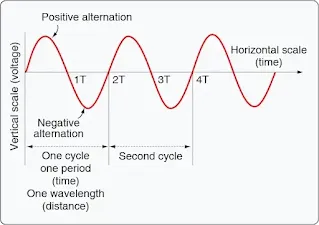Figure 3. Cycle of voltage

One complete cycle is often referred to as the sine wave and said to be 360°. It is typical to start the sine wave where the voltage is zero. The voltage then increases to a maximum positive value, decreases to a value of zero, then increases to a maximum negative value, and again decreases to zero. The cycle repeats until the voltage is no longer available. There are two alternations in a complete cycle: the positive alternation and the negative. It should be noted that the polarity of the voltage reverses for each half cycle. Therefore, during the positive half cycle, the electron flow is considered to be in one direction; during the negative half cycle, the electrons reverse direction and flow the opposite way through the circuit.

### Frequency Defined

The frequency is the number of cycles of AC per second (CPS). The standard unit of frequency measurement is the Hz. [Figure 4] In a generator, the voltage and current pass through a complete cycle of values each time a coil or conductor passes under a north and south pole of the magnet. The number of cycles for each revolution of the coil or conductor is equal to the number of pairs of poles. The frequency, then, is equal to the number of cycles in one revolution multiplied by the number of revolutions per second.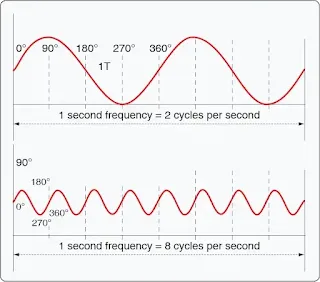Figure 4. Frequency in cycles per second

### Period Defined

The time required for a sine wave to complete one full cycle is called a period (P). A period is typically measured in seconds, milliseconds, or microseconds. [Figure 3] The period of a sine wave is inversely proportional to the frequency. That is to say that the higher the frequency, the shorter the period. The mathematical relationship between frequency and period is given as:

Period
P =
f

Frequency
F =
P

### Wavelength Defined

The distance that a waveform travels during a period is commonly referred to as a wavelength and is indicated by the Greek letter lambda (λ). Wavelength is related to frequency by the formula:

wave speed = wavelength
frequency

The higher the frequency is, the shorter the wavelength is. The measurement of wavelength is taken from one point on the waveform to a corresponding point on the next waveform. [Figure 3] Since wavelength is a distance, common units of measure include meters, centimeters, millimeters, or nanometers. For example, a sound wave of frequency 20 Hz would have wavelength of 17 meters and a visible red light wave of 4.3 × 10 –12 Hz would have a wavelength of roughly 700 nanometers. Keep in mind that the actual wavelength depends on the media through which the waveform must travel.

### Phase Relationships

Phase is the relationship between two sine waves, typically measured in angular degrees. For example, if there are two different alternators producing power, it would be easy to compare their individual sine waves and determine their phase relationship. In Figure 5B, there is a 90° phase difference between the two voltage waveforms. A phase relationship can be between any two sine waves. The phase relationship can be measured between two voltages of different alternators or the current and voltage produced by the same alternator.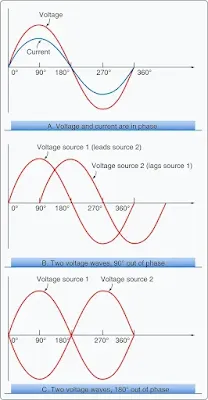Figure 5. In-phase and out-of-phase conditions

Figure 5A shows a voltage signal and a current signal superimposed on the same time axis. Notice that when the voltage increases in the positive alternation that the current also increases. When the voltage reaches its peak value, so does the current. Both waveforms then reverse and decrease back to a zero magnitude, then proceed in the same manner in the negative direction as they did in the positive direction. When two waves are exactly in step with each other, they are said to be in phase. To be in phase, the two waveforms must go through their maximum and minimum points at the same time and in the same direction.

When two waveforms go through their maximum and minimum points at different times, a phase difference exists between the two. In this case, the two waveforms are said to be out of phase with each other. The terms lead and lag are often used to describe the phase difference between waveforms. The waveform that reaches its maximum or minimum value first is said to lead the other waveform. Figure 5B shows this relationship. On the other hand, the second waveform is said to be lagging the first source. When a waveform is said to be leading or lagging, the difference in degrees is usually stated. If the two waveforms differ by 360°, they are said to be in phase with each other. If there is a 180° difference between the two signals, then they are still out of phase even though they are both reaching their minimum and maximum values at the same time. [Figure 5C]

RELATED POSTS